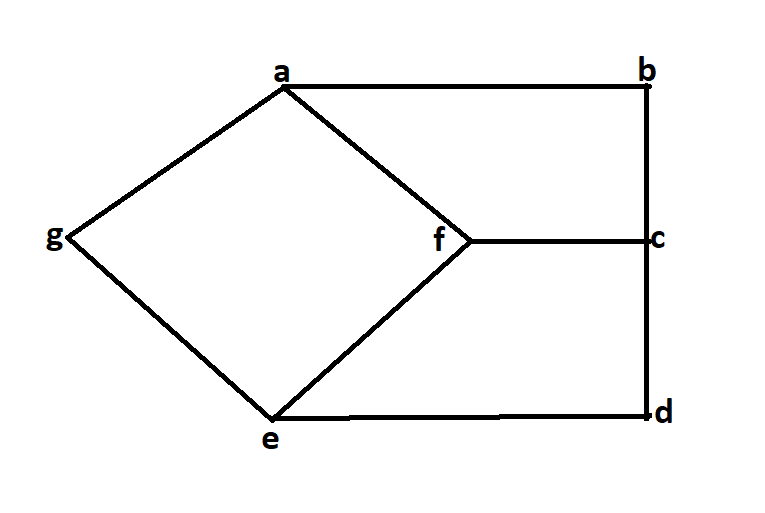GFG App
Open AppBrowser
Continue

# Basic Properties of a Graph

A Graph is a non-linear data structure consisting of nodes and edges. The nodes are sometimes also referred to as vertices and the edges are lines or arcs that connect any two nodes in the graph.

The basic properties of a graph include:

1. Vertices (nodes): The points where edges meet in a graph are known as vertices or nodes. A vertex can represent a physical object, concept, or abstract entity.
2. Edges: The connections between vertices are known as edges. They can be undirected (bidirectional) or directed (unidirectional).
3. Weight: A weight can be assigned to an edge, representing the cost or distance between two vertices. A weighted graph is a graph where the edges have weights.
4. Degree: The degree of a vertex is the number of edges that connect to it. In a directed graph, the in-degree of a vertex is the number of edges that point to it, and the out-degree is the number of edges that start from it.
5. Path: A path is a sequence of vertices that are connected by edges. A simple path does not contain any repeated vertices or edges.
6. Cycle: A cycle is a path that starts and ends at the same vertex. A simple cycle does not contain any repeated vertices or edges.
7. Connectedness: A graph is said to be connected if there is a path between any two vertices. A disconnected graph is a graph that is not connected.
8. Planarity: A graph is said to be planar if it can be drawn on a plane without any edges crossing each other.
9. Bipartiteness: A graph is said to be bipartite if its vertices can be divided into two disjoint sets such that no two vertices in the same set are connected by an edge.

Properties of Graphs are basically used for the characterization of graphs depending on their structures. We defined these properties in specific terms that pertain to the domain of graph theory. In this article, we are going to discuss some properties of Graphs these are as follows:

Distance between two Vertices:

It is basically the number of edges that are available in the shortest path between vertex A and vertex B.If there is more than one edge that is used to connect two vertices then we basically considered the shortest path as the distance between these two vertices.

```Notation used :
d(A, B)
here function d is basically showing the distance between vertex A and vertex B.```
• Let us understand this using an example:•  In the above diagram, let’s try to find the distance between vertices b and d.
```d(b, d)
We can go from vertex b to vertex d in different ways such as
1.ba, af, fe, ed here the d(b, d) will be 4.
2.ba, af, fc, cd here the d(b, d) will be 4.
3.bc, cf, fe, ed here the d(b, d) will be 4.
4.bc, cd here the d(b, d) will be 2.
hence the minimum distance between vertex b and vertex d is 2.```

The eccentricity of a Vertex: Maximum distance from a vertex to all other vertices is considered as the Eccentricity of that vertex.

```Notation used:
e(V)
here e(v) determines the eccentricity of vertex V.```
• Let us try to understand this using following example.```From the above diagram lets try to find the eccentricity of vertex b.
e(b)
d(b, a)=1
d(b, c)=1
d(b, d)=2
d(b, e)=3
d(b, f)=2
d(b, g)=2
Hence the eccentricity of vertex b is 3```

Radius of a Connected Graph: The minimum value of eccentricity from all vertices is basically considered as the radius of connected graph.

```Notation used:
r(G)
here G is the connected graph.```

Let us try to understand this using following example.```From the above diagram:
r(G) is 2.
Because the minimum value of eccentricity from all vertices is 2.```

Diameter of A Connected Graph: Unlike the radius of the connected graph here we basically used the maximum value of eccentricity from all vertices to determine the diameter of the graph.

```Notation used:
d(G)
where G is the connected graph.```
• Let us try to understand this using following example.```From the above diagram:
d(G) is 3.
Because the maximum value of eccentricity from all vertices is 3.```

Central Point and Centre: The vertex having minimum eccentricity is considered as the central point of the graph.And the sets of all central point is considered as the centre of Graph.

```if
e(V)=r(G)
then v is the central point.```
• Let us try to understand this using following example.```In the above diagram the central point will be f.
because
e(f)=r(G)=2
hence f is considered as the central point of graph.
Hence f is also the centre of the graph.```
My Personal Notes arrow_drop_up